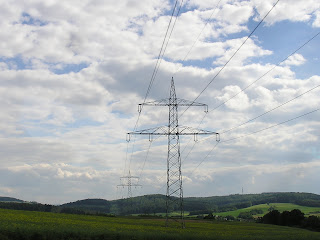Star Connection (Y) In this kind of interconnection, the starting ends or finishing ends (Similar ends) of three coils are connected...

Star Connection (Y)

In this kind of interconnection, the starting ends or finishing ends (Similar ends) of three coils are connected together to form the neutral point. Or
Star Connection is obtained by connecting together similar ends of the three coils, either “Starting” or “finishing”. The other ends are joined to the line wires. The common point is called the neutral or Star Point, which is represented by N. (As shown in fig )
Star Connection is also called Three Phase 4 wires (3-Phase, 4-Wires) system.

If a balance symmetrical load is connected across three phase voltage system in parallel, then the three currents will flow in neutral wire which quantities would be same, but they would be differ by 120° (out of phase), hence the vector sum of these three currents = 0. i.e.
IR + IY + IB = 0   ……………. Victorially

The voltage between any two terminals or Voltage between Line and Neutral (Star Point) is called Phase voltage or Star voltage. And the voltage between two Lines is called Line to Line Voltage or Line Voltage.

Voltage, Current and Power Values in Star Connection (Y)

1.  Line Voltages and Phase Voltages in Star Connection

We know that the Line Voltage between Line 1 and Line 2  is
VRY = VR – VY …. (Vector Difference)
Thus, to find vector of VRY, increase the Vector of VY in reverse direction as shown in the dotted form in the below fig 2. Similarly, on the both ends of vector VR and Vector VY, make perpendicular dotted lines which look like a parallelogram as shown in fig. The Diagonal line which divides the parallelogram into two parts, showing the value of VRY. The angle between VY and VR vectors is 60°.
Hence, if VR = VY = VB = VPH, then
VRY = 2 x VPH x Cos (60°/2)
= 2 x VPH x Cos 30°
= 2 x VPH x (√3/2) …… Since Cos 30° = √3/2
= √3 VPH
Similarly,
VYB = VY – VB
= √3 VPH
And
VBR = VB – VR
= √3 VPH
Hence, it is proved that VRY = VYB = VBR is line voltages Vin Star Connection, Therefore, in Star Connection;
VL = √3 VPH or VL = √3 EPH

It is seen from the fig 2 that;
• Line voltages are 120° apart from each other
• Line voltages are 30° leading from the corresponding phase voltages
• The angle Ф between line currents and respective line voltages are (30°+Ф), i.e. each line current is lagging (30°+Ф) from the corresponding line voltage.

2.   Line Currents and Phase Currents in Star Connection

It is seen from the fig  that each line is in series with individual phase winding, therefore, the value of line current is same as in Phase windings to which the line is connected. i.e.;
• Current in Line 1 = IR
• Current in Line 2 = IY
• Current in Line 3 = IB
Since, the flowing currents in all three lines are same, and the individual current in each line is equal to the corresponding phase current, therefore;
IR = IY = IB = IPH …. The phase current
Line Current = Phase Current
I= IPH
In simple words, the value of Line Current and Phase Current is same in Star Connection.
3.   Power in Star Connection
In a three phase AC circuit, the total True or Active power is the sum of the three phase power. Or the sum of the all three phase powers is the Total Active or True Power.
Hence, total active or true power in a three phase AC system;
Total True or Active Power = 3 Phase Power
Or
P = 3 x VPH x IPH x CosФ                     ….. Eq   … (1)
Good to Know: Where Cos Φ = Power factor = the phase angle between Phase Voltage and Phase Current and not between Line current and line voltage.
We know that the values of Phase Current and Phase Voltage in Star Connection;
I= IPH
VPH = VL /√3     ….. (From VL = √3 VPH)
Putting these values in power eq……. (1)
P = 3 x (VL/√3) x IL x CosФ      …….….      (VPH = VL /√3)
P = √3 x√3 x (VL/√3) x IL x CosФ    ….…   {3 = √3x√3}
P = √3 x VL x IL x CosФ
Hence proved;
Power in Star Connection,
P = 3 x VPH x IPH x CosФ or
P = √3 x VL x IL x CosФ
Similarly,
Total Reactive Power = Q = √3 x VL x IL x SinФ
Good to know: Reactive Power of Inductive coil is taken as Positive (+) and that of a Capacitor as Negative (-).
Also, the total apparent power of the three phases
Total Apparent Power = S = √3 x VL x IL
Or, S = √ (P2 + Q2)

source:electricaltechnology.orgName

BASIC ELECTRICAL,12,BATTERIES,4,CIRCUIT THEORIES,9,CONTROL SYSTEMS,3,DC MOTOR,1,DIGITAL ELECTRONICS,1,DISTRIBUTED GENERATION,2,DISTRIBUTION,6,ELECTRICAL DRIVES,1,ELECTRICAL LAWS,8,ELECTRONICS DEVICES,2,General,7,GENERATION,3,GENERATOR,1,HIGH VOLTAGE,4,ILLUMINATION,1,INDUCTION MOTOR,7,MATERIALS,1,MEASUREMENT,1,MOTOR,1,POWER ELECTRONICS,2,PROJECTS ON INDUCTION MOTOR,1,PROTECTION,1,SMART GRID,3,SWITCHGEAR,4,SYNCHRONOUS MOTOR,1,TRANSFORMER,6,TRANSMISSION,4,
ltr
item
Electrical for Us: Star Connection (Y): Three Phase Power, Voltage & Current Values
Star Connection (Y): Three Phase Power, Voltage & Current Values